Important Questions: Arithmetic Progressions

# Important Questions: Arithmetic Progressions

Test Description

## 10 Questions MCQ Test Mathematics (Maths) Class 10 | Important Questions: Arithmetic Progressions

Important Questions: Arithmetic Progressions for Class 10 2022 is part of Mathematics (Maths) Class 10 preparation. The Important Questions: Arithmetic Progressions questions and answers have been prepared according to the Class 10 exam syllabus.The Important Questions: Arithmetic Progressions MCQs are made for Class 10 2022 Exam. Find important definitions, questions, notes, meanings, examples, exercises, MCQs and online tests for Important Questions: Arithmetic Progressions below.
Solutions of Important Questions: Arithmetic Progressions questions in English are available as part of our Mathematics (Maths) Class 10 for Class 10 & Important Questions: Arithmetic Progressions solutions in Hindi for Mathematics (Maths) Class 10 course. Download more important topics, notes, lectures and mock test series for Class 10 Exam by signing up for free. Attempt Important Questions: Arithmetic Progressions | 10 questions in 10 minutes | Mock test for Class 10 preparation | Free important questions MCQ to study Mathematics (Maths) Class 10 for Class 10 Exam | Download free PDF with solutions
 1 Crore+ students have signed up on EduRev. Have you?
Important Questions: Arithmetic Progressions - Question 1

### The first term and the common difference for the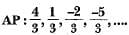are respectively

Important Questions: Arithmetic Progressions - Question 2

### If p, q, r are in AP, then p3 + r3 - 8q3 is equal to

Detailed Solution for Important Questions: Arithmetic Progressions - Question 2

∵ p, q, r are in AP.
∴ 2 q = p + r
⇒ p + r - 2 q = 0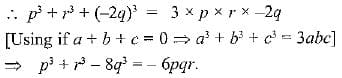Important Questions: Arithmetic Progressions - Question 3

### The next term of the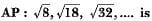Detailed Solution for Important Questions: Arithmetic Progressions - Question 3

The given AP is √8, √18, √32,......... On simplifying the terms, we get: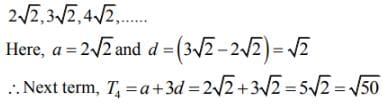Important Questions: Arithmetic Progressions - Question 4

In an AP, if a = 3.5, d = 0, n = 101, then an will be

Detailed Solution for Important Questions: Arithmetic Progressions - Question 4

a101 = 3.5 + 0 (100) = 3.5

Important Questions: Arithmetic Progressions - Question 5

The famous mathematician associated with finding the sum of the first 100 natural numbers is

Detailed Solution for Important Questions: Arithmetic Progressions - Question 5

Johann Friedrich Gauss, he was a German mathematician who find the sum of the first 100 natural number.

Important Questions: Arithmetic Progressions - Question 6

The list of numbers -10, -6, -2, 2, ... is

Important Questions: Arithmetic Progressions - Question 7

The 6th term from the end of the AP: 5, 2, -1 , -4 , . . . , -31 is

Important Questions: Arithmetic Progressions - Question 8

Two APs have the same common difference. The first term of one of these is -1 and that of the other is -8. Then the difference between their 4th terms is

Detailed Solution for Important Questions: Arithmetic Progressions - Question 8

a4 - b4 = (a1 + 3d) - (b1 + 3d)
= a1 b1 = - 1 - (-8) = 7

Important Questions: Arithmetic Progressions - Question 9

Which term of the AP : 21, 42, 63, 84, ... is 210 ?

Important Questions: Arithmetic Progressions - Question 10

If the nth term of an AP is (2n + 1), then the sum of its first three terms is

Detailed Solution for Important Questions: Arithmetic Progressions - Question 10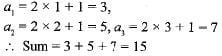## Mathematics (Maths) Class 10

53 videos|369 docs|138 tests
 Use Code STAYHOME200 and get INR 200 additional OFF Use Coupon Code
Information about Important Questions: Arithmetic Progressions Page
In this test you can find the Exam questions for Important Questions: Arithmetic Progressions solved & explained in the simplest way possible. Besides giving Questions and answers for Important Questions: Arithmetic Progressions, EduRev gives you an ample number of Online tests for practice

## Mathematics (Maths) Class 10

53 videos|369 docs|138 tests